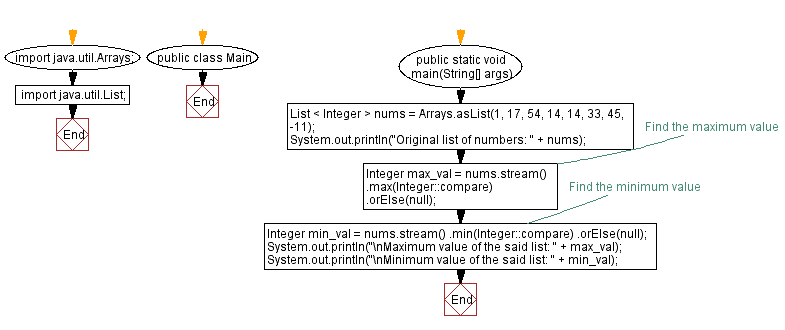﻿ Java Program: Find Maximum and Minimum Values in List of Integers using Streams

# Java Program: Find Maximum and Minimum Values in a List of Integers using Streams

## Java Stream: Exercise-7 with Solution

Write a Java program to find the maximum and minimum values in a list of integers using streams.

Sample Solution:

Java Code:

``````import java.util.Arrays;
import java.util.List;

public class Main {
public static void main(String[] args) {
List < Integer > nums = Arrays.asList(1, 17, 54, 14, 14, 33, 45, -11);
System.out.println("Original list of numbers: " + nums);
// Find the maximum value
Integer max_val = nums.stream()
.max(Integer::compare)
.orElse(null);

// Find the minimum value
Integer min_val = nums.stream()
.min(Integer::compare)
.orElse(null);

System.out.println("\nMaximum value of the said list: " + max_val);
System.out.println("\nMinimum value of the said list: " + min_val);
}
}
```
```

Sample Output:

```Original list of numbers: [1, 17, 54, 14, 14, 33, 45, -11]

Maximum value of the said list: 54

Minimum value of the said list: -11
```

Explanation:

In the above exercise,

We create a list of integers (nums) and use streams to find the maximum value using the max() method and the minimum value using the min() method. The orElse method handles the case when the list is empty. Finally, we print the maximum and minimum values.

Flowchart:Java Code Editor:

Improve this sample solution and post your code through Disqus

What is the difficulty level of this exercise?

Test your Programming skills with w3resource's quiz.

﻿

## Java: Tips of the Day

Converts a string from camelcase:

```public static String fromCamelCase(String input, String separator) {
return input
.replaceAll("([a-z\\d])([A-Z])", "\$1" + separator + "\$2")
.toLowerCase();
}
```

Ref: https://bit.ly/3u09A9E

We are closing our Disqus commenting system for some maintenanace issues. You may write to us at reach[at]yahoo[dot]com or visit us at Facebook